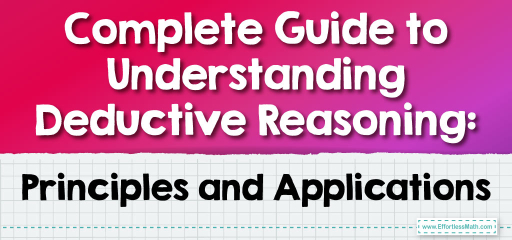# Complete Guide to Understanding Deductive Reasoning: Principles and Applications

Deductive reasoning is a logical process in which conclusions are drawn from a set of given or assumed premises. These conclusions are inherently dependent on the truth and validity of the premises. Often used in formal logic and mathematics, deductive reasoning moves from general principles to specific conclusions. If the premises are true and the logic applied is valid, the conclusion reached will be indisputably true. In geometry, we apply it to prove theorems, validate postulates, and make sense of the shapes and patterns around us. In this guide, we'll demystify deductive reasoning and its pivotal role in geometry. Ready to become a reasoning maestro? Let's begin!## Step-by-step Guide: Deductive Reasoning

What is Deductive Reasoning?
Deductive reasoning, often referred to as top-down logic, starts with a general premise or given truth and examines the possibilities to reach a specific, logical conclusion.

Deductive Reasoning vs. Inductive Reasoning
It’s essential to differentiate between deductive and inductive reasoning:

• Deductive Reasoning: Starts with a general statement or hypothesis and examines the possibilities to reach a specific, logical conclusion.
• Inductive Reasoning: Begins with a set of specific observations or facts and moves to a broader perspective or generalization.

Deductive Reasoning in Geometry
In geometry, deductive reasoning allows us to draw conclusions based on given facts, definitions, postulates, and previously proven theorems. We use logical processes like the Law of Detachment or the Law of Syllogism to derive these conclusions.

Example:
Given: All squares are rectangles.
Given: Figure A is a square.
Conclusion: Figure A is a rectangle. (Derived using deductive reasoning)

### Examples

Example 1:
Given: If it rains, then the grass gets wet.
Given: It is raining.
Solution:
Conclusion: The grass is wet.

Example 2:
Given: If you practice regularly, then you become proficient.
Given: If you become proficient, then you can teach others.
Solution:
Conclusion: If you practice regularly, then you can teach others.

### Practice Questions:

1. Use deductive reasoning to derive a conclusion from the following:
Given: All equilateral triangles have three equal sides.
Given: Triangle ABC is equilateral.
Conclusion: ?
2. Based on the Law of Syllogism:
Given: If a shape has four equal sides, it’s a rhombus.
Given: If a shape is a rhombus, it’s a parallelogram.
Conclusion: ?
3. Determine if deductive reasoning can be applied:
Given: All students in the choir can sing.
Given: John is in the choir.
Conclusion: ?

1. Conclusion: Triangle ABC has three equal sides.
2. Conclusion: If a shape has four equal sides, it’s a parallelogram.
3. Yes, deductive reasoning can be applied. Conclusion: John can sing.

### What people say about "Complete Guide to Understanding Deductive Reasoning: Principles and Applications - Effortless Math: We Help Students Learn to LOVE Mathematics"?

No one replied yet.

X
30% OFF

Limited time only!

Save Over 30%

SAVE $5 It was$16.99 now it is \$11.99